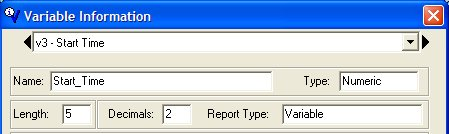# How Do I Calculate the Time Difference Between 2 Variables?

Example: You have 2 variables (Start_Time (V3) and Stop_Time (V4))

The Start_Time & Stop_Time variables must be defined as Numeric with 2 decimal places.When entering data into the Start_Time & Stop_Time variables use a decimal between the hour and minutes.

Example:

10:30 would be entered as 10.30
10:59 would be entered as 10.59

The Calculated time difference variable (V5) can be divided by 60 for minutes or by 3600 for hours.

Example: Time_Diff (V5) would be calculated as

@TIMEDIFF[V3,V4] to return seconds
@TIMEDIFF[V3,V4]/60 to return minutes
@TIMEDIFF[V3,V4]/3600 to return hours

The variable (V5) that calculates the time difference should also have 2 decimal places.Click here to download an example template showing how calculate the time difference between 2 variables.

### Try Quality Window 5 (QW5)

Collect, visualize, analyze and react to your process data so you can increase efficiencies across the manufacturing floor.